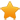Switch Editions?
CancelChannel: CodeSection,代码区,网络安全 - CodeSec
Viewing all articles

# 区块链科普：非对称加密、椭圆曲线加密算法

0
0

RSA算法

RSA中的明文和密钥都是数字。首先，RSA的加密公式为：

y^2 = x^3 + ax + b

y^2 % 23 = x^3 + x % 23

5^2 % 23 = 9^3 + 9 % 23 25 % 23 = 738 % 23 2=2

（由于不是在上数学课，所以这里相应的计算公式也就不再列出了，我们不去深挖细节，只要知道大致原理就好）

y^2 % p = x^3 + 7 % p

p = 2^256 2^32 2^9 2^8 2^7 2^6 2^4 1

K(公钥) = k(私钥) * G(椭圆曲线上的初始点)

k=1E99423A4ED27608A15A2616A2B0E9E52CED330AC530EDCC32C8FFC6A526AEDD